# Resolution of large and small differences in gene expression using models for the Bayesian analysis of gene expression levels and spotted DNA microarrays

## Abstract

### Background

The detection of small yet statistically significant differences in gene expression in spotted DNA microarray studies is an ongoing challenge. Meeting this challenge requires careful examination of the performance of a range of statistical models, as well as empirical examination of the effect of replication on the power to resolve these differences.

### Results

New models are derived and software is developed for the analysis of microarray ratio data. These models incorporate multiplicative small error terms, and error standard deviations that are proportional to expression level. The fastest and most powerful method incorporates additive small error terms and error standard deviations proportional to expression level. Data from four studies are profiled for the degree to which they reveal statistically significant differences in gene expression. The gene expression level at which there is an empirical 50% probability of a significant call is presented as a summary statistic for the power to detect small differences in gene expression.

### Conclusions

Understanding the resolution of difference in gene expression that is detectable as significant is a vital component of experimental design and evaluation. These small differences in gene expression level are readily detected with a Bayesian analysis of gene expression level that has additive error terms and constrains samples to have a common error coefficient of variation. The power to detect small differences in a study may then be determined by logistic regression.

## Background

Spotted DNA microarrays can be used to measure genome-wide gene expression levels in cells of different genotypes, in different developmental states, or within different environments. The precision and accuracy of these measurements depend on the technical performance of the microarray, the degree of replication of the experiment, and the suitability of the model used to analyze the data. A number of models have been advanced for the statistical analysis of experimental designs involving two samples . Two methods, a classical ANOVA method  and a Bayesian method , have been designed for the analysis of experimental designs involving multiple nodes of expression such as genotypes, environments, and developmental states. These analyses yield quantitative results on the expression level of a gene, evaluating data from direct hybridizations as well as data from hybridizations that are informative through transitive inference .

Optimal statistical inference depends upon the choice of model used for analysis. Townsend and Hartl  derived a core model that has been widely used for the estimation of gene expression levels and statistical significance in multifactorial experiments (e.g. ). This model assumed additive small error terms and either estimated error variances for each genotype, environment, or developmental state or estimated a single variance for all genotypes, environments, or developmental states. The ANOVA models of Kerr et al.  and Wolfinger et al.  have also been widely used, and assume multiplicative small error terms. Accordingly, Bayesian models are considered here that incorporate multiplicative error terms. A number of other studies have correlated error variance to raw expression level [2, 1618]. To evaluate the potential effect of this correlation on ratio measurements, models are developed here that constrain the relationship between the error variances and the expression levels to a constant coefficient of variation. Nested error models for spotted DNA microarrays are compared using the Bayesian information criterion for model choice .

The power to detect differences in gene expression using these models is evaluated, and the relationship between the estimated expression level, the number of replicate hybridizations, and the ability to determine the statistical significance of small differences in gene expression is explored both for simulated and empirical data. A summary statistic for determining the fold-resolution detectable as significant in empirical microarray studies is presented.

## Implementation

### Models

#### Model with small additive error effects

The intensity of hybridization of a DNA spot on a microarray is often used as measure of gene expression, but the raw intensity is subject to a number of confounding error terms, such as DNA concentration in a spot and sequence hybridization efficiency. As foreseen by the pioneers of DNA microarray technology, these confounding effects (regardless of their multiplicative or additive nature) are eliminated by consideration of the ratio of hybridization of two samples . The remaining small error terms may be modeled either additively or multiplicatively.

Townsend and Hartl  modeled them additively, deriving a density function for the observed ratio of gene expression in the i th and j th condition, z ij , as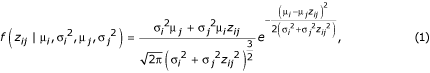where μ i is the expression level in condition i and σ2 i is the variance in condition i. If n conditions or genotypes are under study, direct use of this likelihood function requires estimation of 2n - 1 parameters (n - 1 expression levels plus n variances) for each gene.

One alternative is to constrain the variance so that it is common and equal among nodes of an experimental design , thus reducing the number of parameters requiring estimation to n (i.e. n - 1 expression levels plus one variance). Another alternative is to constrain the variances such that they have a consistent relationship to the expression levels in each node. For example, conditions or genotypes can have a common coefficient of variation (CV) for each gene, ν = σ i / μ i for all i. This alternative also requires the estimation of n parameters (n - 1 expression levels and one CV). It is intuitively motivated by the consideration that larger means for a population are accompanied by larger variances across many phenomena in the sciences . In order to implement a model in which all nodes of an experimental design have a common error CV for each gene, equation 1 may be rewritten, substituting ν2μ i 2 for σ i 2: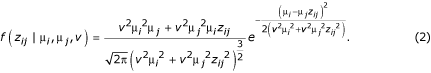Equation 2 can then be used with a prior to construct a Markov chain whose stationary distribution is the posterior distribution of the parameters given the data [22, 23], in all other ways following the algorithm of Townsend and Hartl . The formulation in Equation 2 has additional appeal over Equation 1 when used (as it will be below) within a Markov Chain Monte Carlo (MCMC) analysis. Because values of μ and ν tend to scale similarly across the real line compared to μ and σ2, less tuning of the MCMC jump size may be necessary to achieve a satisfactorily mixed chain.

#### Model with small multiplicative error effects

An alternative to additively modeled error is to model error multiplicatively, such that the post-normalization intensity in one fluorescence channel at a reporter spot is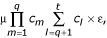where μ is the absolute quantity of mRNA per cell, the c m are spot-associated terms of arbitrary distribution for any a multiplicatively confounding factors, the c l are spot-associated terms of arbitrary distribution for any q-t linearly confounding factors, and ε is a term for small random errors not associated with the spot. The observed ratios of intensities after normalization, y ij , would then be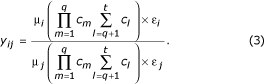Taking the log of both sides,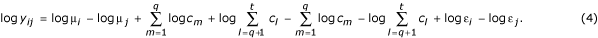The formulation in Equation 4 has some evident similarities to formulations in ANOVA models of gene expression measurement error, where the confounding terms c correspond to the array spot effects identified by Kerr et al.  and Wolfinger et al. , except that the derivation presented here does not assume that these terms are lognormally distributed. Note that these confounding terms are generally not of biological interest and can immediately cancel in equations 3 or 4. Assuming that error terms log ε i and log ε j are composed of many small, unbiased effects, and scaling them so that they are distributed with variances specific to each node, σ i 2 and σ j 2, it follows from equation 3 that the ratio data, z ij , are drawn from a ratio of two lognormal distributions. The numerator is drawn from a lognormal distribution with parameters μ i and σ i 2, and the denominator is drawn from a lognormal distribution with parameters μ j and σ j 2. Just as the difference of two Gaussians is itself Gaussian, the ratio of two lognormals is lognormal, thus the probability density function is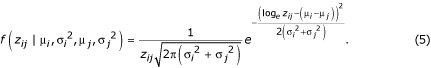Following in all other ways the algorithm of Townsend and Hartl , Equation 5 can then be used with a prior to construct a Markov chain, the stationary distribution of which is the posterior distribution of the parameters given the data. Furthermore, just as with equation 2, all variances for each node may be constrained to be equal or each may be constrained to be linearly proportional to its respective expression level by a single CV. In the latter case, with ν = σ i / μ i = σ j / μ j for all i and all j,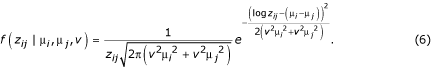### Algorithm

The three-dimensional matrix of ratio results from DNA microarray comparisons, Z, may be constructed, with dimensions i denoting the sample labeled with one fluorophore, j denoting the sample labeled with another, and k denoting the replicate ordinate of that particular dye-labeled comparison. Then, for any continuous structure of comparisons among the nodes of interest, the likelihood density for the parameters μ l and ν l , 1 ≤ l ≤ n, is, by Bayes' rule,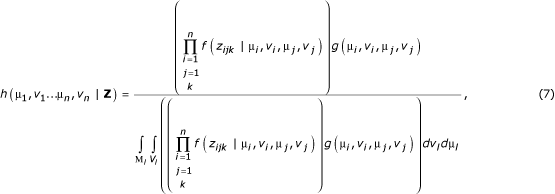where g i , ν i , μ j , ν i ) is the prior distribution of the parameters, and where the probability f(z ijk ) of empty elements in the data matrix Z is properly evaluated as one.

Appropriate informative priors for the variance of microarray data are under investigation [2, 4, 24]. In this paper, a noninformative prior distribution, uniform across positive real numbers, has been used for both the expression levels and for their variances and CVs. The range has been nominally constrained between zero 100, though that upper constraint makes no difference for the datasets examined here. The uniform prior gives the microarray data itself the greatest impact on the inferred expression levels and variances, and implies that credible intervals around parameter estimates (the Bayesian equivalents of classical confidence intervals) are close to those that would be found by maximum likelihood.

Fortunately, we may use the constant denominator of the Bayes' rule formulation (Equation 5) to assert that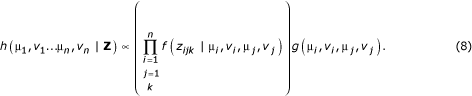Equation 8 may be used to construct a Markov Chain whose stationary distribution is the posterior distribution of the parameters given the data. A vectorof initial error coefficients of variation is chosen arbitrarily, and a vectorof initial expression levels is chosen such that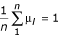at step t = 0. Subsequent values in the chain are determined iteratively by choosing successive proposed values according to an acceptance rule.

Our proposed values are constructed in two separate steps. First, two of the n gene expression level parameters fromare chosen at random. A step size is drawn at random from a triangular distribution centered at zero with range [-Δμ, +Δμ]. The first of the two chosen parameters is incremented by the chosen step size, and the second is decremented by the same quantity, so that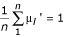is maintained, where the apostrophe indicates a proposed parameter value. In the next iteration, each of the CV parameters inis separately incremented by an amount drawn at random from a triangular distribution with range [-Δν, +Δν] to form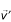. The conjecture is accepted for the next state of the Markov chain if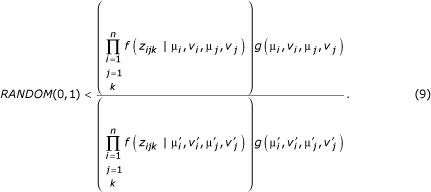Otherwise the original state is retained for the next iteration of the Markov Chain.

These steps are repeated over many generations in order to "burn in" the chain, so that it converges from the initial parameter settings to a stationary distribution. Subsequently, states are sampled from the chain at regular intervals to build a posterior distribution for each parameter, integrated across the probable states of all other parameters. All analyses in this paper were performed with 20,000 generations of burn-in, followed by 200,000 generations during which the chain was sampled every 20 generations to construct the posterior distribution. Runs using multiple starting vectorsandwere performed and always converged to the same, unimodal posteriors. Results reported here were the outcomes of Markov chains started with the elements ofall equal to one, and started with the elements ofequal to 0.2. Step sizes, Δμ and Δν, were tuned for each gene so that acceptance ratios for each parameter update were in the efficient and well-mixed range, (0.15, 0.50) . If acceptance ratios for either parameter jump were less than 0.15 or greater than 0.5, the chain was run again with a better-tuned jump size, until acceptable ratios for both parameters were obtained. In this way, there is no alteration of the jump size during any run. There is only the evaluation of pilot Markov chains to optimize jump size.

### Output

This implementation of these models can accommodate complex experimental designs, where a number of genotypes, environments, and developmental time points are examined. Within this framework, missing data (e.g. excluded single spots, or even missing hybridizations) do not require special consideration or a change in methodology; credible intervals and P values reflect accurately the degree to which the data informs each estimate. This software allows the quantitative information on gene expression levels from microarrays to be thoroughly analyzed and carefully considered in assessing the biological effects of genetic or environmental differences of cellular state.

Output from the software implementation is in the form of a tab-delimited text file with one header row. Each row thereafter displays the results for a single gene, including columns with: the estimate of expression level for each node (the median of the posterior distribution); the additions and subtractions to make 95% upper and lower bounds on that estimate; the stationary acceptance rates for the Monte Carlo steps for that gene; and the posterior probabilities (P values) for whether the expression level of a gene in each expression node is greater, or lesser, than the expression level of that gene in each other expression node.

### Evaluation

#### Nested model choice

The common variance (AV, MV) and common CV (AC, MC) models are both nested within their respective general unconstrained variance model (AU, MU). The same number of parameters is estimated in both of the nested models. They differ only in how the estimated variances are constrained with relation to the estimated expression levels. Whether the nested models are appropriate compared to the general model may be assessed using the Bayesian Information Criterion , which is to choose the model m that maximizes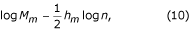where M m is the maximum likelihood of model m, h m is the number of parameters estimated in the model, and n is the number of observations.

#### Tests of power

Simulated data sets have an advantage over real data sets, in that true gene expression levels for simulated data are known. Data sets were simulated to ensure that methods introduced here yielded appropriate results when data was derived from a number of reasonable and proposed distributions for gene expression data. For simulated data sets, six ratio measurements were drawn 1400 times from each of five distributions. The simulated distributions were sampled from by the following procedures. For the ratio of normal distributions with a single variance term among all nodes of the experimental design, ratios were created by the division of a random variable drawn from a Normal distribution by another random variable drawn from a Normal distribution, then discarded if outside the range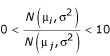. For the ratio of normal distributions with a single CV term among all nodes, ratios were created by the division of a random variable drawn from a Normal distribution by another random variable drawn from a Normal distribution, then discarded if outside the range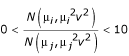. For the lognormal distribution, ratios were drawn directly from log N(μ, σ2) or log N(μ, μ2ν2). For the simulation of data from the Gamma distribution and the Cauchy distribution, parameters were chosen such that the means of the distributions were the same as the intended true expression level. Ratios drawn from the Cauchy distribution were discarded if they were below zero or above ten.

For each distribution, 1000 measurements of gene expression level were simulated where both samples had the same expression level, and one hundred measurements were simulated for ratios of expression level of 1.1, 1.25, 1.5, and 2. Variance and CV parameters for all the above distributions simulated expression levels were set at the average values inferred from the dataset of Townsend et al.  under additive models. Note that, although parameters of each distribution were generally chosen so that the variances of the ratio output of each distribution would be similar, no attempt was made to make higher moments than the mean identical. Therefore, the relevant comparisons are between analysis methods on a given simulated dataset, and frequencies of significance calling are not directly comparable across simulated datasets.

#### Logistic regressions

Power to detect a difference in gene expression depends critically on the true factor of fold-difference between samples. A continuous logistic function,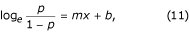describing the probability of detection of statistical significance, p, of simulated log2 factors of difference in gene expression, x, was parameterized with an intercept, b, and slope, m, by logistic regression. The same regression was performed on real data by substituting estimates of the factor of difference in gene expression level for known factors of difference, thus providing a profile of the power of an experiment to detect differences in gene expression. A useful metric for such an analysis is the factor of difference in gene expression level that has a fifty percent chance of being identified as significant. Herein, this is referred to as the GEL50, for the Gene Expression Level at which there is a 50 percent chance of detection of statistical significance.

## Results

The general and nested models were implemented on two independent published data sets large enough to estimate parameters within the general model [10, 26]. The Bayesian Information Criterion (BIC)  was used for model choice. For both datasets examined, the nested models had considerably higher BIC values than the general models, regardless of the kind of error model (Table 1), indicating that the nested models, with fewer parameters, are preferable.

Computation time for analysis of published data sets varied across models (Table 1). Computation using additive models (AV, AC, AU) was more rapid than computation using multiplicative models. Regardless of whether small error terms were modeled as additive or multiplicative, constrained CV models (AC, MC) were faster than constrained variance (AV, MV) or general unconstrained (AU, MU) models. Furthermore, the relative ranks of these models in terms of speeds of computation, without exception, remained as above in all analyses of simulated datasets.

In the analysis of data simulated as a ratio of two normal distributions, model AC exhibited the greatest power to detect true differences in gene expression (Figure 1). Model AV performed nearly as well, whereas the unconstrained model (AU) was dramatically less powerful across expression levels (Figure 1A,1C). Among multiplicative models, model MC exhibited the greatest power, followed by models MV and MU, which were barely distinguishable (Figure 1B,1C). Regardless of whether data was simulated with equal error variances in each sample (Figure 1A,1B) or error standard deviations proportional to the expression level in each sample (Figure 1C,1D), assuming the constrained coefficient of variance model yielded the greatest power to detect differences in gene expression level.

In the analysis of data simulated as a ratio of two lognormal distributions, model AC again exhibited the greatest power to detect true differences in gene expression (Figure 2). Model AV performed nearly as well, whereas the unconstrained model (AU) was considerably less powerful across expression levels (Figure 2A,2C). Among multiplicative models, model MC exhibited the greatest power, followed by models MV and MU, which were indistinguishable (Figure 2B,2C). Regardless of whether data was simulated with equal variances in each node (Figure 2A,2B) or standard deviations proportional to the expression level in each node, assuming the constrained coefficient of variance model yielded the greatest power to detect differences in gene expression level.

In the analysis of ratio data simulated from Cauchy and Gamma distributions, model AC again was found to exhibit the greatest power to detect true differences in gene expression (Figure 3). Model AV performed slightly less well, whereas the unconstrained model (AU) was considerably less powerful across expression levels (Figure 2A,2C). Multiplicative models all demonstrated similar power. Model MC exhibited the greatest power, followed by models MV and MU, which were indistinguishable (Figure 2B,2C). Regardless of the distribution of ratio measurements, assuming the constrained coefficient of variance model yielded the greatest power to detect differences in gene expression level.

Higher power to detect true differences, although important in practice for the purpose of choice of model in an experimental study, does not indicate a better fit to the data. This is made clear by comparing Figure 1A with Figure 1C, and by comparing Figure 2B with Figure 2D. If the increased power to detect true differences in gene expression were solely due to a better fit to the data, then analysis via model AV would outperform analysis via model AC in Figure 1A, and analysis via model MV would outperform analysis via model MC in Figure 2B. Thus, constraining the nodes of the experimental design to a single error CV yields greater power than constraining the nodes to single error variance, regardless of which analysis model fits the data better.

The power to detect differences in gene expression as a continuous function of the log2 factor of difference in gene expression for the simulated data shown in Figure 1C that was analyzed by model AC is plotted in Figure 4A. The smaller the true difference in gene expression, the less likely it is to be identified as significantly different between two nodes in an experimental design. The lack of resolution for detection of small differences with any given experimental design is a characteristic of experimental measurement, whether the variances or coefficients of variation are constrained or unconstrained. It persists across all models examined.

In comparison to performing logistic regression of the frequency of positive calls versus true differences in gene expression level, a logistic regression of the frequency of positive calls versus the estimates of gene expression level derived from analysis of the simulated data may be performed (Figure 4B). Stochasticity in the estimation due to small sample size causes dispersion of the values along the abscissa. Nevertheless, this regression on estimates of gene expression level shows the same decreasing probability of significant call with decreasing gene expression difference between nodes of an experimental design.

Fortunately, the regression in Figure 4B may be performed not just on simulated data, but also on data from experimental studies. The power to detect differences in gene expression of various magnitudes between nodes of experimental designs is plotted for four published studies in Figure 5. A useful summary of the power is the factor of gene expression level at which there is a 50% frequency of a significant call (GEL50). Alexandre et al.  compared yeast at log-phase growth with and without 30 minutes of exposure to ethanol. The experimental design incorporated two nodes and three hybridizations. The GEL50 for their study was 2.8-fold (Figure 5A). Lyons et al.  studied zinc regulation in yeast. Their experimental design included nine reported hybridizations on six nodes. The comparison of gene expression levels between wild type and zap1 strains at log-phase growth in low zinc media is plotted here. The GEL50 for this comparison was 1.65-fold (Figure 5B). A study by Sudarsanam et al.  comprised two data sets examining the effects of swi1 and snf2 mutations in rich media and in minimal media on gene expression. The regressions for comparisons of wild type and snf2 strains at log-phase growth in the two media are plotted in Figure 5C. The right-hand curve is drawn from analysis of the experiment in rich media, which was restricted to just five hybridizations among three nodes. The GEL50 for this comparison was 1.91-fold. The left-hand curve is drawn from analysis of the experiment in minimal medium. Six microarray hybridizations were performed between the three nodes, and GEL50 = 1.49-fold. This is a direct demonstration of the increased resolution achieved with increased replication. Townsend et al.  examined gene expression levels in natural isolates of wine yeast at log-phase growth. Ten microarray hybridizations were performed among four nodes. The GEL50 for the comparison of two of these isolates, M1-2 and M2-8, was 1.56-fold. Across all of these experiments, increased replication yielded greater resolution of the statistical significance of small differences in gene expression.

## Discussion

Distinguishing the optimal models to use for the analysis of replicated spotted DNA microarray data is important. Optimized models will yield qualitatively more accurate lists of significantly differently expressed genes, and quantitatively more precise resolution of smaller differences in gene expression. The Bayesian Information Criterion for model selection can be used to choose between models that invoke distinct error variances or coefficients of variation for each node as characterized by genotype, environment, and developmental state, and the nested models that invoke a single variance or CV for all nodes. The values of the BIC for the relatively small studies examined here (Table 1) clearly support analysis with the nested models that invoke a single variance or CV.

In addition to direct assessment of the fit of the model to the data, power to detect known differences may guide model choice. Generally, the ranking of the power of models was consistent regardless of the distribution used to simulate the data (Figures 1, 2, and 3). For unconstrained models that estimate a variance or CV term for each node, the analysis model incorporating an assumption of small multiplicative error terms (model MU) had greater power to detect differences than the analysis model incorporating an assumption of small additive error terms (model AU). Nested models that invoke a single variance or CV had higher power to detect known differences when the analysis model incorporated an assumption of small additive error terms (Equation 1, i.e. models AC and AV had higher power than model AU.) Among analysis models incorporating an assumption of small multiplicative error terms (Equation 2), only one of the two nested models, model MC, had consistently higher power than the unconstrained model. Overall, a model incorporated an assumption of small additive error terms and a single error CV for all nodes (model AC) had the greatest power to detect differences in gene expression level. In practice, model AC was also the fastest computationally (Table 1), perceptibly requiring fewer tuning steps to find an appropriate jump size for the generation of posterior distributions by Markov Chain Monte Carlo.

If variances are generally proportional to their expression levels, then the constrained CV models (AC and MC) pertain. A linear regression of the estimated coefficients of variation to their respective expression levels should have positive slope. Specifically, regressions on the datasets here typically have positive slope (y = ~ 0.4x + c) and are highly statistically significant, although the data exhibit considerable scatter and thus poor correlation (r2 ~ 0.04). These data sets are barely large enough to estimate error variances in a gene-by-gene manner using the general model. Future experimental data with greater replication, analyzed by the general model, will yield higher precision estimates of the error variances and thus better resolution of this question.

When the nominal false positive rate α = 0.05, all models have an actual false positive rate that is moderately to considerably less than 0.05, averaging 0.02 (Figures 1, 2, and 3). These false positive rates are close enough to zero that precise estimation of the frequency requires extensive computation, but generally, the false positive rate was slightly higher with the more powerful models. The slightly lower than nominal false-positive rate is due to the flat prior on the error variance or error CV, that is slightly too permissive of large estimates of the variance and of the CV. Further investigation into the use of a more informative prior distribution (such as the gamma distribution, e.g. ), is called for, requiring larger studies with greater replication.

Prediction of the number of replicates required for statistical significance testing of microarray data is theoretically possible [29, 30], by making specific assumptions about the error variances and the level of gene expression difference of interest. Here, empirical examination of the power to detect significant differences at different gene expression levels in different studies (Figure 5) has the potential to simply and rapidly convey vital evaluative feedback about the design, replication, and technical performance of a set of hybridizations. All three of these factors contribute to the ability of a microarray study to resolve small factors of difference in gene expression between nodes in an experimental design. This ability to resolve differences can be summarized by a single, intuitive parameter: the gene expression level at which there is a 50% frequency of significant calls (GEL50). Note, however, that the regressions in Figure 5, like the volcano plots of Wolfinger et al. , show genes with a broad range of estimated expression levels that are significant, and genes with a broad range of estimated expression levels that are not significant. Therefore, analyses that invoke a fold-change threshold as an indication of significance should be avoided.

From the datasets analyzed here, it is clear that increased replication leads to greater resolution of small differences in gene expression (Figure 5). This small number of studies, of varying technical quality, does not warrant a strictly quantitative empirical formula for the GEL50 based on the number of nodes in the experimental design and the number of replicate hybridizations. However, a very crude rule of thumb based upon examination of the quality and resolution of these and other datasets is that the GEL50 resolution of a study is of the form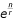, where n is the number of nodes in the design and r is the total number of hybridizations performed. The studies examined here all contained replicated comparisons, and, in accord with MIAME standards , reported ratio results from each hybridization. Future analyses of a range of additional studies that also report results of each hybridization for each gene will have the potential to reveal a more accurate and precise prediction of power using more sources of information about the quality of the microarray hybridizations and about the optimal design of multifactorial experiments .

Increased power to detect differences in gene expression, consequent to better analysis, better replication, or better technical performance, identifies more significant differences in gene expression of genes with smaller and smaller true expression differences. These small differences in gene expression are not only present [10, 32], they are relevant to the evolution of gene regulation  and to organismal function and phenotype [32, 33]. Transcription factors, for instance, may have enormous impact on cellular function with minimal changes in expression level [34, 35]. The detection of the differential expression of transcription factors is often a major goal of many microarray studies. Therefore, understanding the resolution of difference in gene expression that is detectable as significant is a vital component of experimental design and evaluation.

## Availability and requirements

Project name: Bayesian Analysis of Gene Expression Level (BAGEL)

Operating system(s): MacOS 9, MacOS X, Windows and Linux.

Other requirements: none

## Abbreviations

Models used will be abbreviated with a two-letter acronym. The first letter indicates (A)dditive or (M)ultiplicative error:

and the second letter indicates a general (U)nconstrained variance model, a constrained (V)ariance model, or a constrained (C)oefficient of variation model. Thus, the AV and AC models are nested within the AU model, while the MV and MC models are nested within the MU model. With n nodes in the experimental design, the AU and MU models both have 2n - 1 parameters (n - 1 expression levels plus n variances), and the AV, AC, MV, and MC models all have n parameters (n- 1 expression levels, plus 1 variance or CV).

## References

1. Ideker T, Thorsson V, Siegel AF, Hood LE: Testing for differentially-expressed genes by maximum-likelihood analysis of microarray data. Journal of Computational Biology 2000, 7: 805–817. 10.1089/10665270050514945

2. Baldi Pierre, Long Anthony: A Bayesian framework for the analysis of microarray expression data: regularized t-test and statistical inferences of gene changes. Bioinformatics 2001, 17: 509–519. 10.1093/bioinformatics/17.6.509

3. Theilhaber Joachim, Bushnell Steven, Jackson Amanda, Fuchs Rainer: Bayesian estimation of fold-changes in the analysis of gene expression: the PFOLD algorithm. Journal of Computational Biology 2001, 8: 585–614. 10.1089/106652701753307502

4. Tseng George C., Oh Min-Kyu, Rohlin Lars, Liao James C., Wong Wing Hung: Issues in cDNA microarray analysis: quality filtering, channel normalization, models of variations and assessment of gene effects. Nucleic Acids Research 2001, 29: 2549–2557. 10.1093/nar/29.12.2549

5. Kerr M. Kathleen, Martin Mitchell, Churchill Gary A.: Analysis of variance for gene expression microarray data. Journal of Computational Biology 2000, 7: 819–837. 10.1089/10665270050514954

6. Kerr M. Kathleen, Churchill Gary A.: Experimental design for gene expression microarrays. Biostatistics 2001, 2: 183–201. 10.1093/biostatistics/2.2.183

7. Wolfinger Russell D., Gibson Greg, Wolfinger Elizabeth D., Bennett Lee, Hamadeh Hisham, Bushel Pierre, Afshari Cynthia, Paules Richard S.: Assessing gene significance from cDNA microarray expression data via mixed models. Journal of Computational Biology 2001, 8: 625–637. 10.1089/106652701753307520

8. Townsend Jeffrey P., Hartl Daniel L.: Bayesian analysis of gene expression levels: statistical quantification of relative mRNA level across multiple treatments or samples. Genome Biology 2002, 3: research0071.1–71.16. 10.1186/gb-2002-3-12-research0071

9. Townsend Jeffrey P.: Multifactorial experimental design and the transitivity of ratios with spotted DNA microarrays. BMC Genomics 2003, 4: 41. 10.1186/1471-2164-4-41

10. Townsend Jeffrey P., Cavalieri Duccio, Hartl Daniel L.: Population genetic variation in genome-wide gene expression. Molecular Biology and Evolution 2003, 20: 955–963. 10.1093/molbev/msg106

11. Meiklejohn Colin D., Parsch John, Ranz JM, Hartl Daniel L.: Rapid evolution of male-biased gene expression in Drosophila. Proceedings of the National Academy of Sciences - USA 2003, 100: 9894–9899. 10.1073/pnas.1630690100

12. Ranz JM, Castillo-Davis CI, Meiklejohn Colin D., Hartl Daniel L.: Sex-dependent gene expression and evolution of the Drosophila transcriptome. Science 2003, 300: 1742–1745. 10.1126/science.1085881

13. Silverman Neal, Zhou Rui, Ehrlich Rachel, Hunter Mike, Bernstein Erik, Schneider David, Maniatis Tom: Immune activation of NF-kB and JNK requires Drosophila TAK1. The Journal of Biological Chemistry 2003, 278: 48928–48934. 10.1074/jbc.M304802200

14. Whitfield Charles W., Cziko Anne-Marie, Robinson Gene E.: Gene expression profiles in the brain predict behavior in individual honey bees. Science 2003, 302: 296–299. 10.1126/science.1086807

15. Grozinger Christina M., Sharabash Noura M., Whitfield Charles W., Robinson Gene E.: Pheromone-mediated gene expression in the honey bee brain. Proceedings of the National Academy of Sciences - USA 2003, 100: 14519–14525. 10.1073/pnas.2335884100

16. Rocke David M., Durbin Blythe: A model for measurement error for gene expression arrays. Journal of Computational Biology 2001, 8: 557–569. 10.1089/106652701753307485

17. Mutch DM, Berger A, Mansourian R, Rytz A, Roberts MA: The limit fold change model: a practical approach for selecting differentially expressed genes from microarray data. BMC Bioinformatics 2002, 3: 17. 10.1186/1471-2105-3-17

18. Durbin Blythe, Rocke David M.: Estimation of transformation parameters for microarray data. Bioinformatics 2003, 19: 1360–1367. 10.1093/bioinformatics/btg178

19. Schwarz Gideon: Estimating the dimension of a model. The Annals of Statistics 1978, 6: 461–464.

20. Eisen MB, Brown PO: DNA arrays for analysis of gene expression. Methods Enzymol 1999, 303: 179–205. 10.1016/S0076-6879(99)03014-1

21. Sokal Robert R., Rohlf F. James: Biometry. 3rd Edition New York, W. H. Freeman and Company 1995, 887.

22. Metropolis Nicholas, Rosenbluth Arianna W., Rosenbluth Marshall N., Teller Augusta H., Teller Edward: Equation of state calculations by fast computing machines. Journal of Chemical Physics 1953, 21: 1087–1092.

23. Hastings WK: Monte Carlo sampling methods using Markov chains and their applications. Biometrika 1970, 57: 97–109.

24. Newton MA, Kendziorski CM, Richmond CS, Blattner FR, Tsui KW: On differential variability of expression ratios: improving statistical inference about gene expression changes from microarray data. Journal of Computational Biology 2001, 8: 37–52. 10.1089/106652701300099074

25. Gelman A, Roberts GO, Gilks WR: Efficient Metropolis jumping rules. Bayesian Statistics 5 (Edited by: Bernardo JM, Berger JO, Dawid AP and Smith AFM). Oxford University Press 1996, 599–607.

26. Sudarsanam Priya, Iyer Vishwanath R., Brown Patrick O., Winston Fred: Whole-genome expression analysis of snf/swi mutants of Saccharomyces cerevisiae. Proceedings of the National Academy of Sciences - USA 2000, 97: 3364–3369. 10.1073/pnas.050407197

27. Alexandre H, Ansanay-Galeote V, Dequin S, Blondin B: Global gene expression during short-term ethanol stress in Saccharomyces cerevisiae. FEBS Letters 2001, 498: 98–103. 10.1016/S0014-5793(01)02503-0

28. Lyons Thomas J, Gasch Audrey P, Gaither L. Alex, Botstein David, Brown Patrick, Eide David: Genome-wide characterization of the Zap1p zinc-responsive regulon in yeast. Proceedings of the National Academy of the Sciences 2000, 97: 7957–7962. 10.1073/pnas.97.14.7957

29. Wernisch Lorenz: Can replication save noisy microarray data? Comparative and Functional Genomics 2002, 3: 372–374. 10.1002/cfg.196

30. Pan Wei, Lin Jizhen, Le Chap T: How many replicates are required to detect gene expression changes in microarray experiments? A mixture model approach. Genome Biology 2002, 3: research0022.1. 10.1186/gb-2002-3-5-research0022

31. Brazma Alvis, Hingamp Pascal, Quackenbush John, Sherlock Gavin, Spellman Paul, Stoeckert Chris, Aach John, Ansorge Wilhelm, Ball Catherine A., Causton Helen C., Gaasterland Terry, Glenisson Patrick, Holstege Frank C. P., Kim Irene F., Markowitz Victor, Matese John C., Parkinson Helen, Robinson Alan, Sarkans Ugis, Schulze-Kremer Steffen, Stewart Jason, Taylor Ronald, Vilo Jaak, Vingron Martin: Minimum information about a microarray experiment (MIAME)–toward standards for microarray data. Nature Genetics 2001, 29: 365–371. 10.1038/ng1201-365

32. Rockman Matthew V., Wray Gregory A.: Abundant raw material for cis-regulatory evolution in humans. Molecular Biology and Evolution 2002, 19: 1991–2004.

33. Yan Hai, Dobbie Zuzana, Gruber Stephen B., Markowitz Sanford, Romans Kathy, Giardiello Francis M., Kinzler Kenneth W., Vogelstein Bert: Small changes in expression affect predisposition to tumorigenesis. Nature Genetics 2002, 30: 25–26. 10.1038/ng799

34. Gibson G: Epistasis and pleiotropy as natural properties of transcriptional regulation. Theor Popul Biol 1996, 49: 58–89. 10.1006/tpbi.1996.0003

35. Doebley John, Lukens Lewis: Transcriptional regulators and the evolution of plant form. The Plant Cell 1998, 10: 1075–1082. 10.1105/tpc.10.7.1075

## Acknowledgements

Thanks to Takao Kasuga, Alison Galvani, and Betty Gilbert for comments on the manuscript. JPT was supported while performing this work by a Research Fellowship from the Miller Institute for Basic Research in Science.

## Author information

Authors

### Corresponding author

Correspondence to Jeffrey P Townsend.

## Authors’ original submitted files for images

Below are the links to the authors’ original submitted files for images.

## Rights and permissions

Reprints and Permissions

Townsend, J.P. Resolution of large and small differences in gene expression using models for the Bayesian analysis of gene expression levels and spotted DNA microarrays. BMC Bioinformatics 5, 54 (2004). https://doi.org/10.1186/1471-2105-5-54

• Accepted:

• Published:

• DOI: https://doi.org/10.1186/1471-2105-5-54

### Keywords

• Gene Expression Level
• Nest Model
• Unconstrained Model
• Jump Size
• Error Standard Deviation